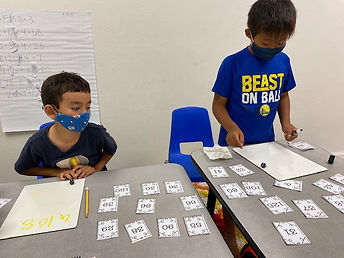## Ms. Kylie

### Target 1​

###### Lesson Type:

Review

Number Operation

:

Computation

Solve multi-step problems within 1,000 (addition, subtraction, multiplication, and division).

###### 1:

Solve problems that have more than one operation.

###### 2:

Understand that the presence of parenthesis in an arithmetic equation signals for this part of the problem to be solved first.

###### 3:

Understand that the order of operations must be followed to evaluate expressions.

###### 4:

Define PEMDAS – Parenthesis, Exponents, Multiplication, Division, Addition, Subtraction.

5th

###### Vocabulary:

Order of Operation, PEMDAS

Activities:

-Students reviewed solving multi-step equations. They used PEMDAS to solve the equations.

-Students played an order of operations game. They were given a deck of cards with numbers and rolled a dice to get there 3 numbers. They needed to use their knowledge of order of operations to create an equation with the 3 numbers to try to get the answer to be on one of the cards. The winner of the game is the one who was able to collect the most cards.

-Students were given word problems and needed to write a multi-step equation to solve the problem.### Home Exploration

###### Guiding Questions:## Absent Students:

### Target 2

:

###### Vocabulary:

Activities:### Home Exploration

###### Guiding Questions:### Target 3

:

###### Vocabulary:

Activities:### Home Exploration